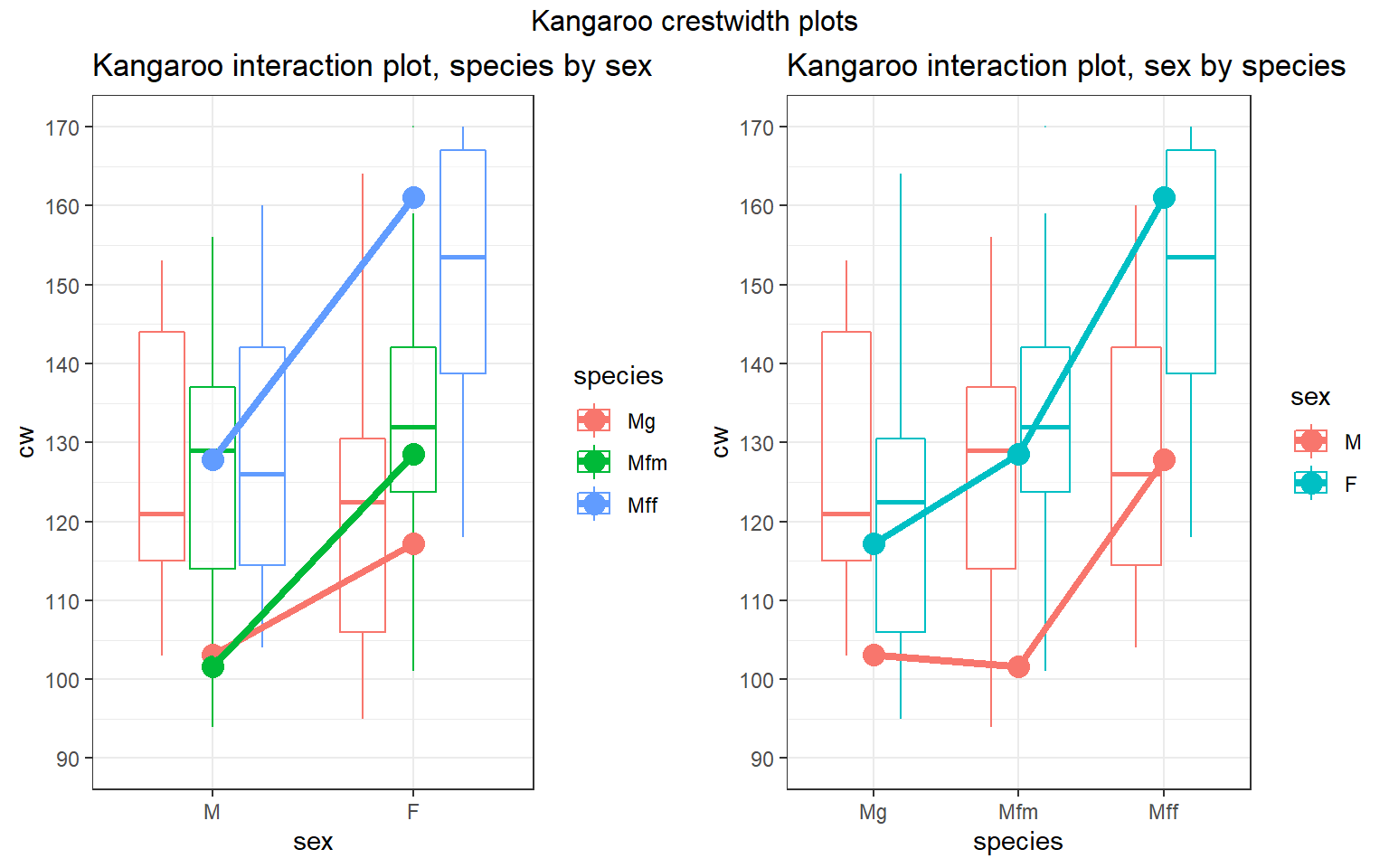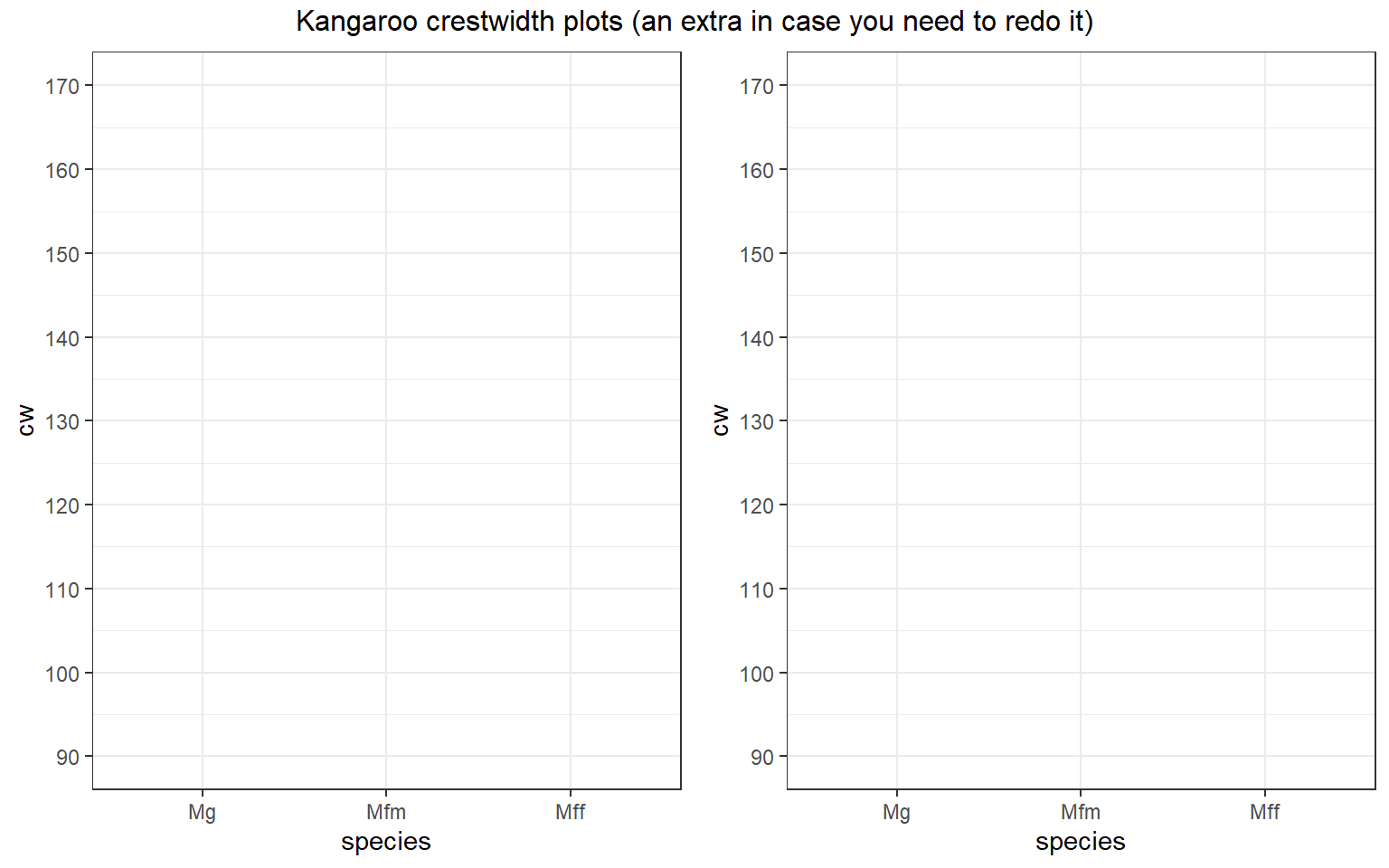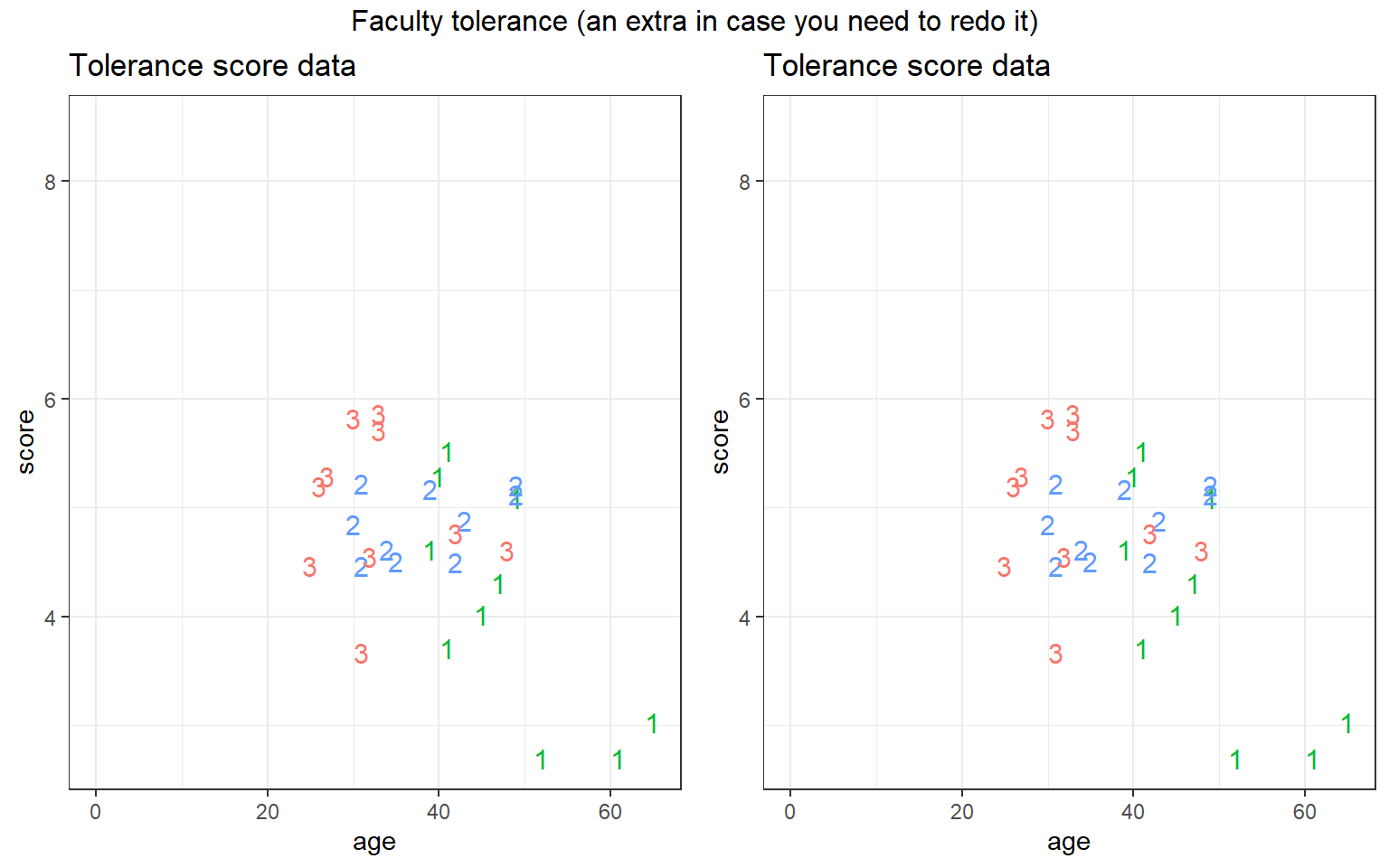This assignment is to be printed and hand-written.

In my opinion, some of the most important skills in modeling are:

• writing down a model using indicator variables,
• interpretting model coefficients,
• solving for the predicted value for any combination of predictors, and
• plotting the fitted model.

This assignment applies these skills to two-way factor models (ADA2 Chapter 5) and ANCOVA models with one factor and one continuous predictor (ADA2 Chapter 7).

# 1 1. Two-way main-effect model: Kangaroo crest width

Recall these data, results, and the model from Week 05.

library(tidyverse)

kang <-
, na = c("", ".")
) %>%
# subset only our columns of interest
select(
sex, species, cw
) %>%
# make dose a factor variable and label the levels
mutate(
sex     = factor(sex    , labels = c("M","F"))
, species = factor(species, labels = c("Mg", "Mfm", "Mff"))
)

str(kang)
Classes 'spec_tbl_df', 'tbl_df', 'tbl' and 'data.frame':    148 obs. of  3 variables:
$sex : Factor w/ 2 levels "M","F": 1 1 1 1 1 1 1 1 1 1 ...$ species: Factor w/ 3 levels "Mg","Mfm","Mff": 1 1 1 1 1 1 1 1 1 1 ...
$cw : num 153 141 144 116 120 188 149 128 151 103 ... # A tibble: 6 x 3 sex species m <fct> <fct> <dbl> 1 M Mg 103. 2 M Mfm 102. 3 M Mff 128. 4 F Mg 117. 5 F Mfm 128. 6 F Mff 161lm_cw_x_s <- lm( cw ~ sex + species , data = kang ) # parameter estimate table summary(lm_cw_x_s)  Call: lm(formula = cw ~ sex + species, data = kang) Residuals: Min 1Q Median 3Q Max -94.456 -19.746 1.553 23.478 90.216 Coefficients: Estimate Std. Error t value Pr(>|t|) (Intercept) 97.784 6.039 16.193 < 2e-16 *** sexF 24.673 6.070 4.064 7.89e-05 *** speciesMfm 4.991 7.460 0.669 0.505 speciesMff 34.280 7.383 4.643 7.66e-06 *** --- Signif. codes: 0 '***' 0.001 '**' 0.01 '*' 0.05 '.' 0.1 ' ' 1 Residual standard error: 36.91 on 144 degrees of freedom Multiple R-squared: 0.2229, Adjusted R-squared: 0.2067 F-statistic: 13.77 on 3 and 144 DF, p-value: 6.057e-08 ## 1.1(2 p) Write the fitted model equation. Use the parameter estimate table above to write out the fitted model equation. Use indicator function notation for categorical variables. First determine what each sex and species number is. The equation looks like: $$\hat{y} = [\text{terms}]$$. ### 1.1.1 Solution ## 1.2(2 p) Separate model equations. For each combination of species and sex, write the model. ### 1.2.1 Solution Sex Species Fitted Model M Mg $$\hat{y}=$$ \ M Mfm $$\hat{y}=$$ \ M Mff $$\hat{y}=$$ \ F Mg $$\hat{y}=$$ \ F Mfm $$\hat{y}=$$ \ F Mff $$\hat{y}=$$ ## 1.3(1 p) Plot the observed and fitted values. Use symbols/colors/labels to distinguish between the observed and predicted values and clearly identify the species/sex combinations. Use the minimum about of labeling to make it clear. ### 1.3.1 Solution# 2 2. ANCOVA model: Faculty political tolerances A political scientist developed a questionnaire to determine political tolerance scores for a random sample of faculty members at her university. She wanted to compare mean scores adjusted for the age for each of the three categories: full professors (coded 1), associate professors (coded 2), and assistant professors (coded 3). The data are given below. Note the higher the score, the more tolerant the individual. Below we will fit and interpret a model to assess the dependence of tolerance score on age and rank. (We will assess model fit in a later assignment.) tolerate <- read_csv("http://statacumen.com/teach/ADA2/worksheet/ADA2_WS_11_tolerate.csv") %>% mutate( rank = factor(rank) # set "3" as baseline level , rank = relevel(rank, "3") ) str(tolerate) Classes 'spec_tbl_df', 'tbl_df', 'tbl' and 'data.frame': 30 obs. of 3 variables:$ score: num  3.03 4.31 5.09 3.71 5.29 2.7 2.7 4.02 5.52 4.62 ...
$age : num 65 47 49 41 40 61 52 45 41 39 ...$ rank : Factor w/ 3 levels "3","1","2": 2 2 2 2 2 2 2 2 2 2 ...

## 2.1(2 p) Write the fitted model equation.

Note in the code what the baseline rank is.

lm_s_a_r_ar <-
lm(
score ~ age * rank
, data = tolerate
)
summary(lm_s_a_r_ar)

Call:
lm(formula = score ~ age * rank, data = tolerate)

Residuals:
Min       1Q   Median       3Q      Max
-1.34746 -0.28793  0.01405  0.36653  1.07669

Coefficients:
Estimate Std. Error t value Pr(>|t|)
(Intercept)  5.42706    0.98483   5.511 1.15e-05 ***
age         -0.01321    0.02948  -0.448   0.6580
rank1        2.78490    1.51591   1.837   0.0786 .
rank2       -1.22343    1.50993  -0.810   0.4258
age:rank1   -0.07247    0.03779  -1.918   0.0671 .
age:rank2    0.03022    0.04165   0.726   0.4751
---
Signif. codes:
0 '***' 0.001 '**' 0.01 '*' 0.05 '.' 0.1 ' ' 1

Residual standard error: 0.6378 on 24 degrees of freedom
Multiple R-squared:  0.5112,    Adjusted R-squared:  0.4093
F-statistic:  5.02 on 5 and 24 DF,  p-value: 0.002748

Use the parameter estimate table above to write out the fitted model equation. Use indicator function notation for categorical variables. The equation looks like: $$\hat{y} = [\text{terms}]$$.

## 2.2(2 p) Separate model equations.

There’s a separate regression line for each faculty rank.

### 2.2.1 Solution

Rank Fitted Model
1 $$\hat{y}=$$
\
2 $$\hat{y}=$$
\
3 $$\hat{y}=$$

## 2.3(1 p) Plot the fitted regression lines.

Use symbols/colors/labels to distinguish between the observed and predicted values and clearly identify the rank. Use the minimum about of labeling to make it clear. I recommend plotting each line by evaluating two points then connecting them, for example, by evaluating at age=0 and age=50.

### 2.3.1 Solution